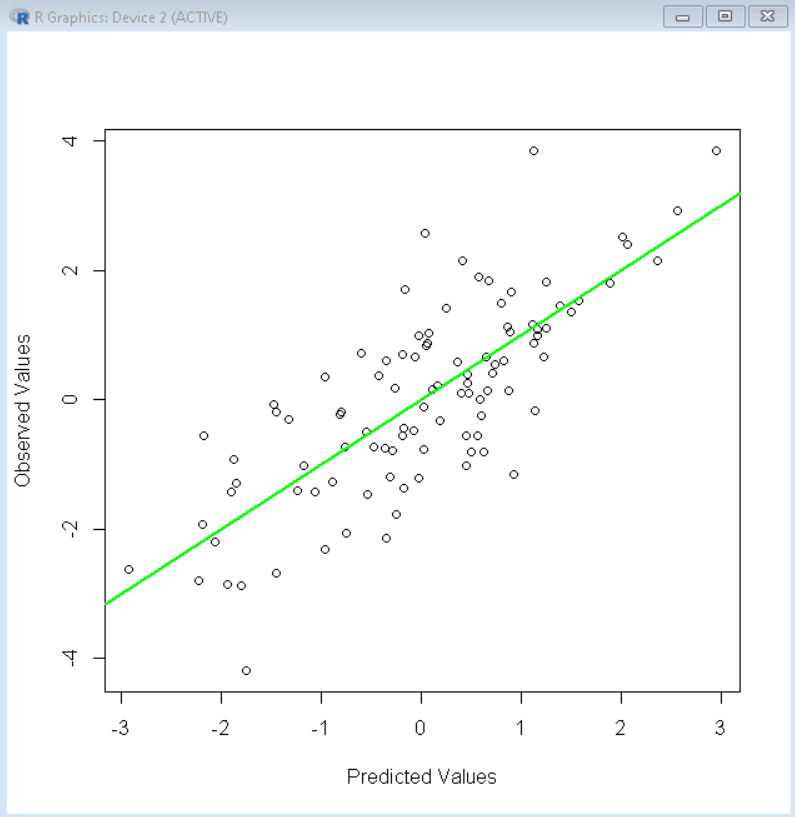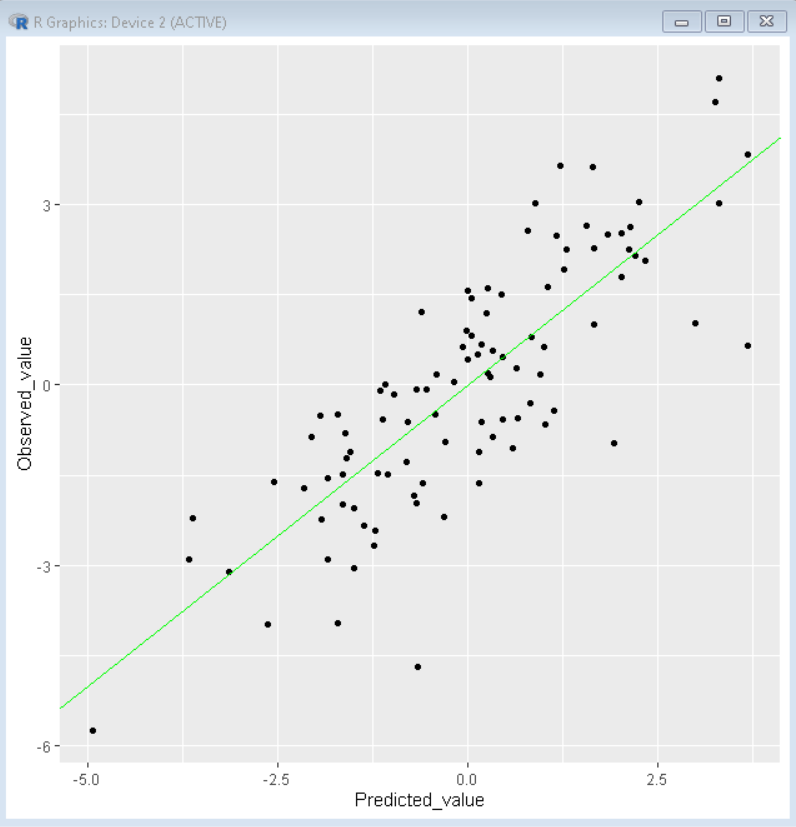# How to Plot Predicted Values in R?

• Last Updated : 19 Dec, 2021

In this article, we will discuss how to plot predicted values in the R Programming Language.

A linear model is used to predict the value of an unknown variable based on independent variables using the technique linear regression. It is mostly used for finding out the relationship between variables and forecasting. The lm() function is used to fit linear models to data frames in the R Language. We plot the predicted actual along with actual values to know how much both values differ by, this helps us in determining the accuracy of the model. To do so, we have the following methods in the R Language.

## Method 1: Plot predicted values using Base R

To plot predicted value vs actual values in the R Language, we first fit our data frame into a linear regression model using the lm() function. The lm() function takes a regression function as an argument along with the data frame and returns linear model. Then we can use predict() function to use that linear model to predict values for any given data point. We will then plot a scatter plot between the predicted value and actual by using the plot() function and then add linear diagonal line using the abline() function to visualize the difference between predicted and actual values.

Syntax:

linear_model <- lm( regression_function, df)

plot( predict(linear_model), df\$y)

abline(a = 0, b = 1)

where,

• regression_function: determines the function on which linear model has to be fitted.
• df: determines the data frame that is used for prediction.
• y: determines the y-axis variable.

Example: Here, is a plot of actual values vs predicted values using a linear regression model using the Base R methods.

## R

 `# create sample data frame ` `x <- ``rnorm``(100) ` `y <- ``rnorm``(100) + x ` `sample_data <- ``data.frame``(x, y) ` ` `  `# fit data to a linear model ` `linear_model <- ``lm``(y~x, sample_data ) ` ` `  `# plot predicted values and actual values ` `plot``(``predict``(linear_model), sample_data\$y, ` `     ``xlab = ``"Predicted Values"``, ` `     ``ylab = ``"Observed Values"``) ` `abline``(a = 0, b = 1, lwd=2, ` `       ``col = ``"green"``)`

Output:## Method 2: Plot predicted values using the ggplot2 package

To plot predicted value vs actual values in the R Language using the ggplot2 package library, we first fit our data frame into a linear regression model using the lm() function. The lm() function takes a regression function as an argument along with the data frame and returns a linear model. Then we make a data frame that contains the predicted value and actual value for plotting. to get predicted values, we can use predict() function to use that linear model to predict values for any given data point. We will then plot a scatter plot between the predicted value and actual by using the ggplot() function with the geom_point() function and then add a linear diagonal line using the geom_abline() function to visualize the difference between predicted and actual values.

Syntax:

linear_model <- lm( regression_function, df)

plot_data <- data.frame( predicted_data = predict(linear_model), actual_data= df\$y )

ggplot( plot_data, aes( x=predicted_data, y=actual_data ) ) + geom_point()+ geom_abline(intercept =0, slope=1)

where,

• regression_function: determines the function on which linear model has to be fitted.
• df: determines the data frame that is used for prediction.
• y: determines the y-axis variable.

Example: Here, is a plot of actual values vs predicted values using a linear regression model using the ggplot2 package.

## R

 `# create sample data frame ` `x1 <- ``rnorm``(100) ` `x2 <- ``rnorm``(100) ` `y <- ``rnorm``(100) + x1 + x2 ` `sample_data <- ``data.frame``(x1, x2, y) ` ` `  `# fit data to a linear model ` `linear_model <- ``lm``(y~x1+x2, sample_data ) ` ` `  `# load library ggplot2 ` `library``(ggplot2) ` ` `  `# create dataframe with actual and predicted values ` `plot_data <- ``data.frame``(Predicted_value = ``predict``(linear_model),   ` `                       ``Observed_value = sample_data\$y) ` ` `  `# plot predicted values and actual values ` `ggplot``(plot_data, ``aes``(x = Predicted_value, y = Observed_value)) + ` `                  ``geom_point``() + ` `                 ``geom_abline``(intercept = 0, slope = 1, color = ``"green"``)`

Output:My Personal Notes arrow_drop_up
Recommended Articles
Page :